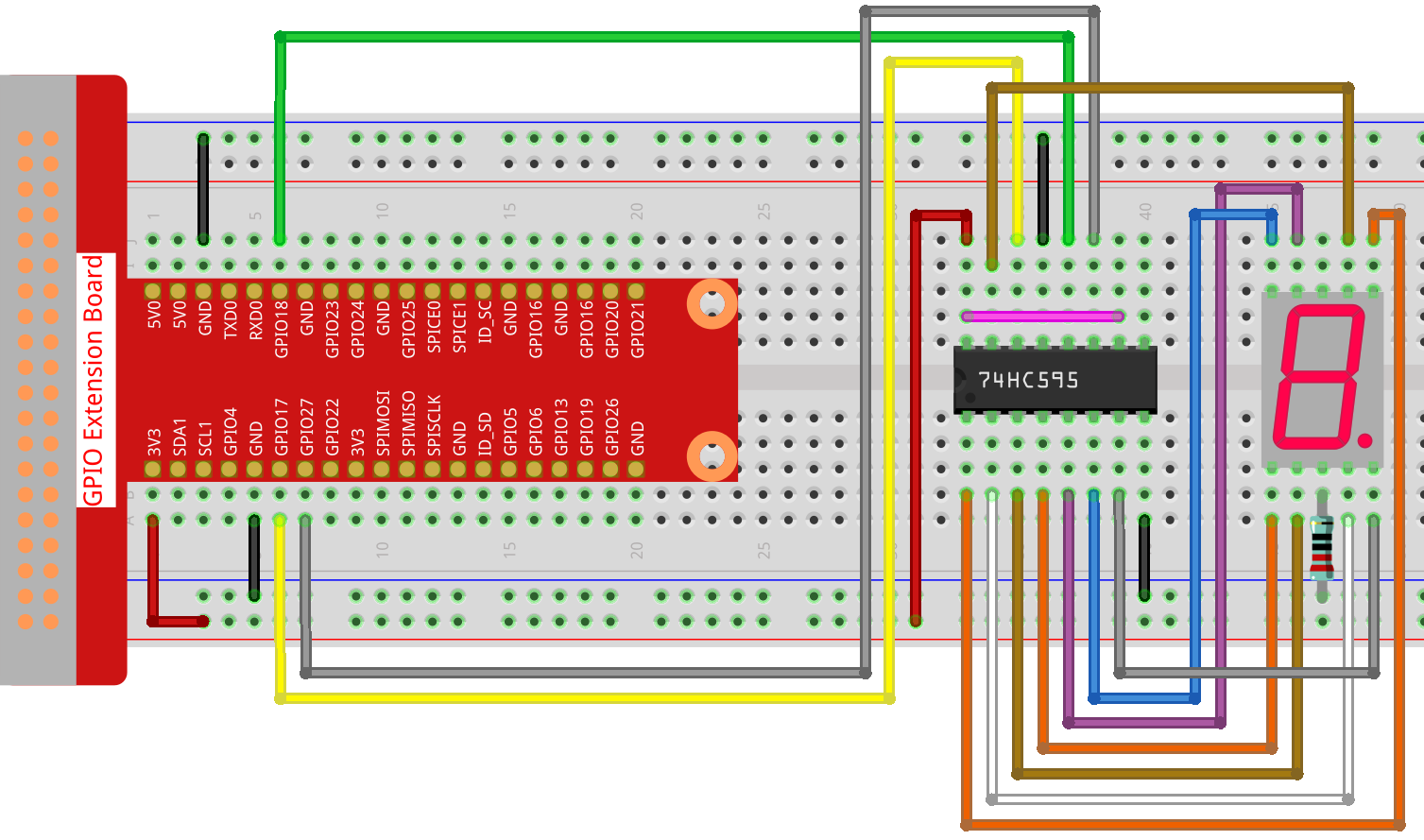# Show Number¶

In this project, we use processing to drive a 7-segment display to show a figure from 0 to 9 and A to F.

WiringSketch

```import processing.io.*;

int SDI=17;   //serial data input
int RCLK=18;  //memory clock input(STCP)
int SRCLK =27;   //shift register clock input(SHCP)

int[] SegCode= {0x3f,0x06,0x5b,0x4f,0x66,0x6d,0x7d,0x07,0x7f,0x6f,0x77,0x7c,0x39,0x5e,0x79,0x71};

void hc595_shift(int dat){
int i;

for(i=0;i<8;i++){
int n=(0x80 & (dat << i));
if ( n==0){
GPIO.digitalWrite(SDI, 0);
} else {
GPIO.digitalWrite(SDI, 1);
}
GPIO.digitalWrite(SRCLK, 1);
delay(1);
GPIO.digitalWrite(SRCLK, 0);
}

GPIO.digitalWrite(RCLK, 1);
delay(1);
GPIO.digitalWrite(RCLK, 0);
}

void setup() {
size(400, 200);
frameRate(10);

GPIO.pinMode(SDI, GPIO.OUTPUT);
GPIO.pinMode(RCLK, GPIO.OUTPUT);
GPIO.pinMode(SRCLK, GPIO.OUTPUT);

GPIO.digitalWrite(SDI, 0);
GPIO.digitalWrite(RCLK, 0);
GPIO.digitalWrite(SRCLK, 0);

fill(0,25,88);
textAlign(CENTER,CENTER);
textSize(height*0.8);
}

void draw() {

background(255);
int number = (frameCount%100)/10;
text(number, width/2, height/2);
hc595_shift(SegCode[number]);
}
```

How it works?

Import `processing.io.*` and use the GPIO function library to control the digital tube pins.

Define array `SegCode = {0x3f,0x06,0x5b,0x4f,0x66,0x6d,0x7d,0x07,0x7f,0x6f,0x77,0x7c,0x39,0x5e,0x79,0x71}` which represents a segment code array from 0 to F in Hexadecimal (Common cathode).

`setup()` function sets the three pins SDI,RCLK and SRCLK as output, and the initial data as 0.

`hc595_shift(int dat)` function is used to shift the `SegCode` to 74HC595.

```void hc595_shift(int dat){
int i;

for(i=0;i<8;i++){
int n=(0x80 & (dat << i));
if ( n==0){
GPIO.digitalWrite(SDI, 0);
} else {
GPIO.digitalWrite(SDI, 1);
}
GPIO.digitalWrite(SRCLK, 1);
delay(1);
GPIO.digitalWrite(SRCLK, 0);
}

GPIO.digitalWrite(RCLK, 1);
delay(1);
GPIO.digitalWrite(RCLK, 0);
}
```

`n=(0x80 & (dat << i))` means to shift dat to the left by `i` bits and then do the `&` operation with 0x80.

The rule of `&` operation is that when both sides of `&` are 1, the result is 1, otherwise the result is 0.

For example, we assume dat=0x3f,i=2(0011 1111 << 2 shift to 1111 1100), then 1111 1100 & 1000 0000 (0x80)) = 1000 0000.

At last assign the dat data to SDI(DS) by bits.

`digitalWrite(SRCLK, 1)` when SRCLK generates a rising edge pulse from 0 to 1, the data will be transferred from the DS register to the shift register;

`digitalWrite(RCLK, 1)` when RCLK generates a rising edge pulse from 0 to 1, the data will be transferred from the shift register to the storage register.

```fill(0,25,88);
textAlign(CENTER,CENTER);
textSize(height*0.8);
```

The `fill()` function used in `setup()` can fill the text color, `textAlign(CENTER,CENTER)` is used to center the text, `textSize(height*0.8)` change the text height to 0.8 times the original. These functions can customize the text style displayed on the processing

```void draw() {

background(255);
int number = (frameCount%100)/10;
text(number, width/2, height/2);
hc595_shift(SegCode[number]);
}
```

The `frameCount` is a seed, which is related to `frameRate`. By default `frameRate` is 60, which means that `frameCount` will accumulate 60 times per second.

Then we can let processing and 7-segment display to show the figure from 0 to 9 and A to F simultaneously.